# 1 6 Solving Inequalities Solving Inequalities Solving inequalities

• Slides: 8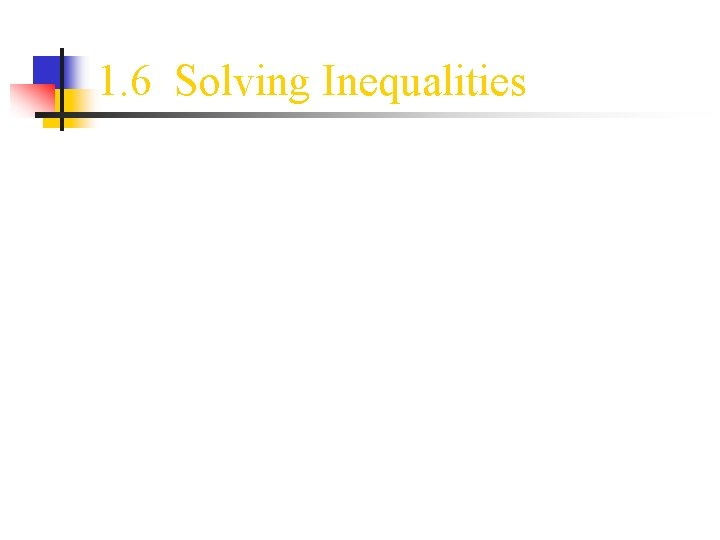1. 6 Solving Inequalities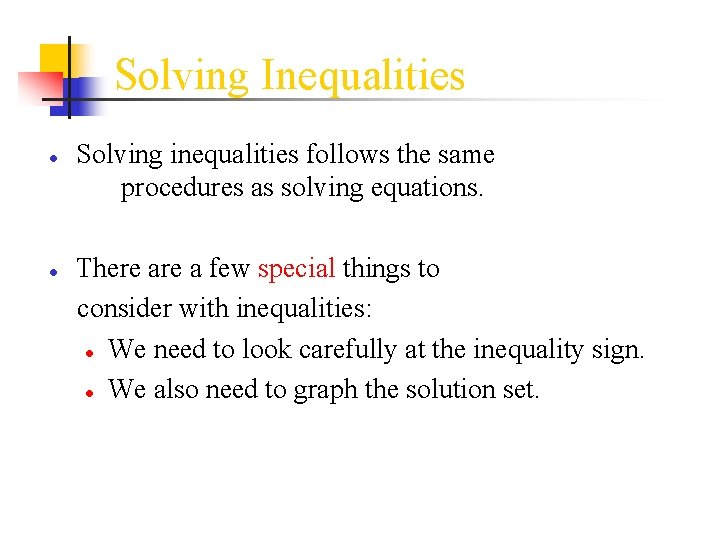Solving Inequalities ● Solving inequalities follows the same procedures as solving equations. ● There a few special things to consider with inequalities: ● We need to look carefully at the inequality sign. ● We also need to graph the solution set.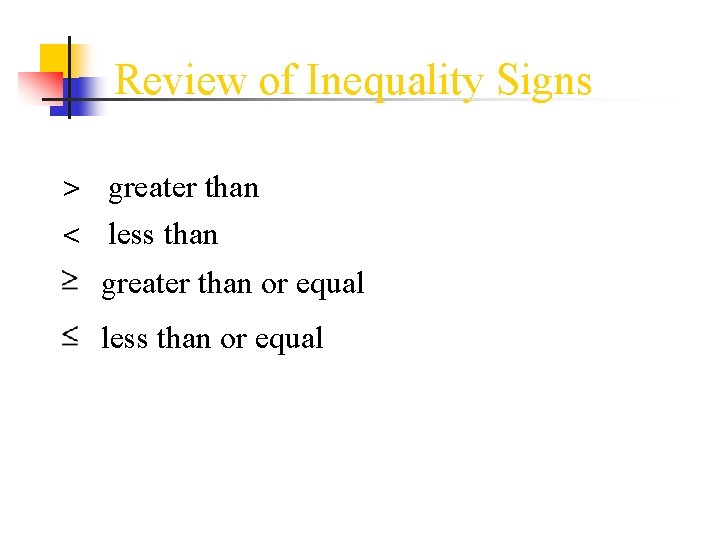Review of Inequality Signs > greater than < less than greater than or equal less than or equal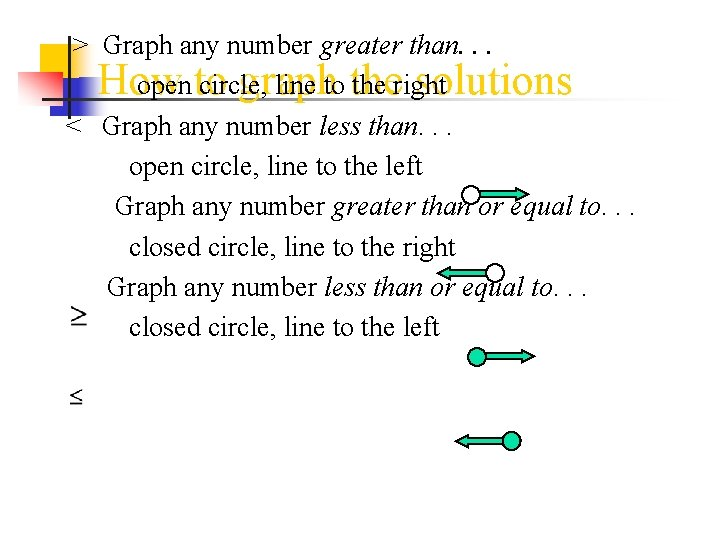> Graph any number greater than. . . How graph solutions open to circle, line to the right < Graph any number less than. . . open circle, line to the left Graph any number greater than or equal to. . . closed circle, line to the right Graph any number less than or equal to. . . closed circle, line to the left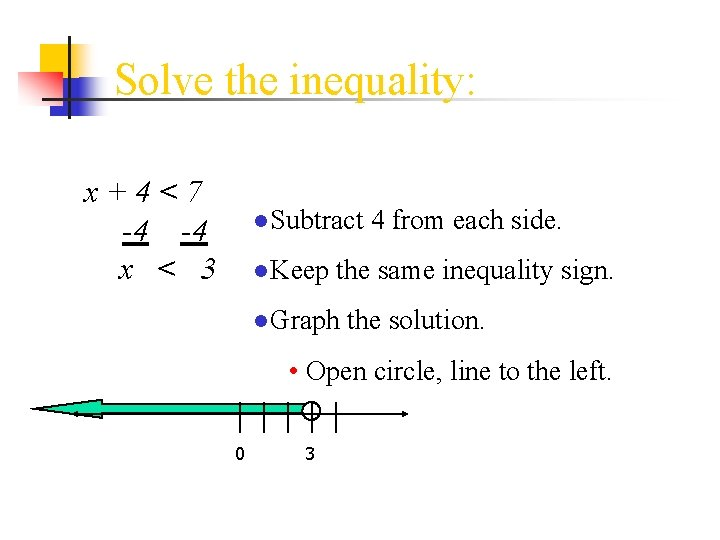Solve the inequality: x+4<7 -4 -4 x < 3 ●Subtract 4 from each side. ●Keep the same inequality sign. ●Graph the solution. • Open circle, line to the left. 0 3There is one special case. ● Sometimes you may have to reverse the direction of the inequality sign!! ● That only happens when you multiply or divide both sides of the inequality by a negative number.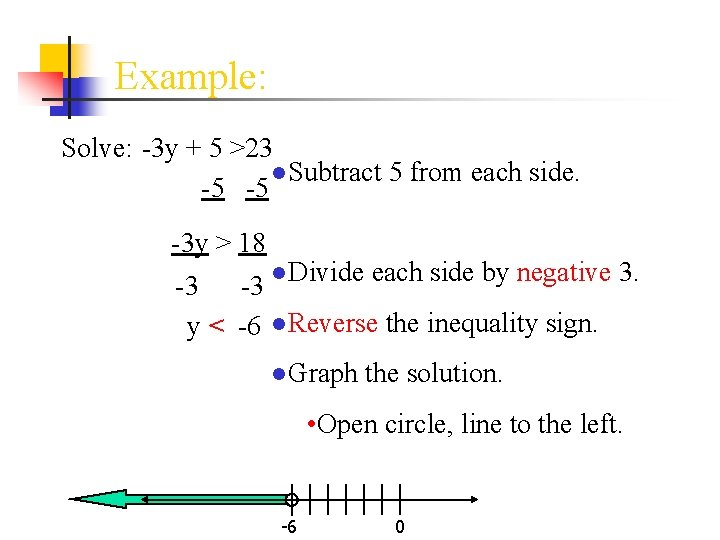Example: Solve: -3 y + 5 >23 ●Subtract 5 from each side. -5 -5 -3 y > 18 -3 -3 ●Divide each side by negative 3. y < -6 ●Reverse the inequality sign. ●Graph the solution. • Open circle, line to the left. -6 0Try these: ● Solve 2 x+3>x+5 ● Solve - c - 11>23 ● Solve 3(r-2)<2 r+4